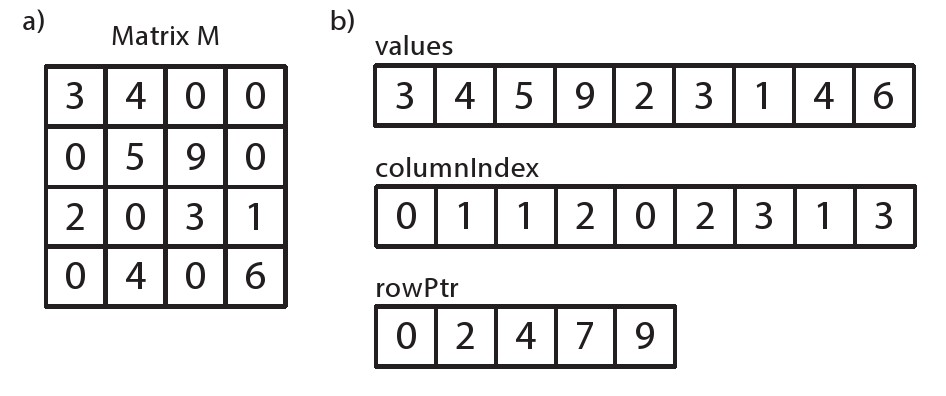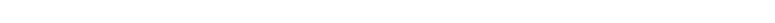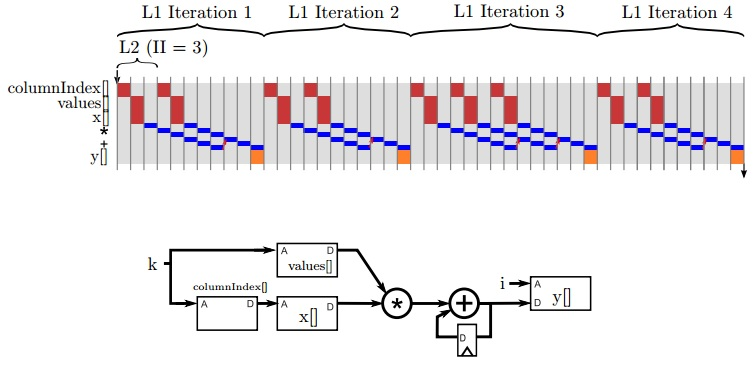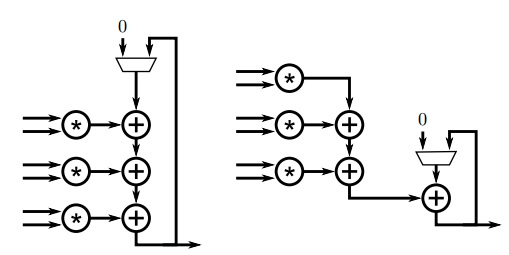# 稀疏矩阵向量乘

FPGA矩阵计算并行算法与结构(知网)

## 稀疏矩阵向量乘法的优化（1）对矩阵A做行列分块处理（2）自适应分块存储结构（3）减少下标存储的冗余

（4）多线程和NUMA特性

Memory #0是CPU #0的本地内存，Memory #1是CPU #1的本地内存，它们有各自独立的内存带宽。CPU #0访问Memory #1需要经过内部总线（在Intel的架构中叫QPI总线），这个总线的带宽一般小于内存带宽。另外如果要访问的数据只集中在一颗CPU的本地内存中，那么只能利用一个NUMA node的内存带宽，这就限制了系统的总体吞吐。## FPGA上的稀疏矩阵向量乘稀疏矩阵向量乘法

### 6.1 背景图 6.1: M是一个4x4矩阵，用两种方式表示：同"密集"矩阵一样存在二维数组之中；作为稀疏矩阵，以行压缩存储的形式保存，行压缩存储是一种由3个数组组成的数据结构。

  1 2 3 4 5 6 7 8 9 10 11 12 13 14  #include "spmv.h" void spmv(int rowPtr[NUM_ROWS+1], int columnIndex[NNZ], DTYPE values[NNZ], DTYPE y[SIZE], DTYPE x[SIZE]){ L1: for (int i = 0; i < NUM_ROWS; i++) { DTYPE y0 = 0; L2: for (int k = rowPtr[i]; k < rowPtr[i+1]; k++) { #pragma HLS unroll factor=8 #pragma HLS pipeline y0 += values[k] * x[columnIndex[k]]; } y[i] = y0; } }图6.2: 主体代码演示了系数矩阵向量乘（SpMV）y=M.x的计算。采用CRS的方式，通过rowPt*、columnIndex 和 value 保存矩阵M。第一个for循环通过迭代访问每一行，第二个for循环访问每一列，实现矩阵M中非0元素和向量中对应的元素相乘并保存值在向量y中。

### 6.2 基本实现

  1 2 3 4 5 6 7 8 9 10 11  #ifndef __SPMV_H__ #define __SPMV_H__ const static int SIZE = 4; // SIZE of square matrix const static int NNZ = 9; //Number of non-zero elements const static int NUM_ROWS = 4;// SIZE; typedef float DTYPE; void spmv(int rowPtr[NUM_ROWS+1], int columnIndex[NNZ], DTYPE values[NNZ], DTYPE y[SIZE], DTYPE x[SIZE]); #endif // __MATRIXMUL_H__ not defined图6.3： spmv函数和激励的头文件

### 6.3 测试平台

  1 2 3 4 5 6 7 8 9 10 11 12 13 14 15 16 17 18 19 20 21 22 23 24 25 26 27 28 29 30 31 32 33 34 35 36 37  #include "spmv.h" #include void matrixvector(DTYPE A[SIZE][SIZE], DTYPE *y, DTYPE *x) { for (int i = 0; i < SIZE; i++) { DTYPE y0 = 0; for (int j = 0; j < SIZE; j++) y0 += A[i][j] * x[j]; y[i] = y0; } } int main(){ int fail = 0; DTYPE M[SIZE][SIZE] = { {3,4,0,0},{0,5,9,0},{2,0,3,1},{0,4,0,6} }; DTYPE x[SIZE] = {1,2,3,4}; DTYPE y_sw[SIZE]; DTYPE values[] = {3,4,5,9,2,3,1,4,6}; int columnIndex[] = {0,1,1,2,0,2,3,1,3}; int rowPtr[] = {0,2,4,7,9}; DTYPE y[SIZE]; spmv(rowPtr, columnIndex, values, y, x); matrixvector(M, y_sw, x); for(int i = 0; i < SIZE; i++) if(y_sw[i] != y[i]) fail = 1; if(fail == 1) printf("FAILED\n"); else printf("PASS\n"); return fail; }图6.4 ： 一个简单spmv函数的简单测试平台。测试平台生成了一个用例，并且计算矩阵的向量乘法通过稀疏矩阵乘法（spmv）和非系数矩阵乘法(matrixvector)。

### 6.4 指定循环的属性

spmv函数能正常工作的前提条件是什么？证明给定的前提条件，矩阵中每个非0元素实是不是在对应一次内层循的执行？

loop_tripcount 引导能帮助设计者对函数的性能有个原始的估计。这样能比较相同的函数通过使用不同的directives或者对代码本身重构。但是，这不能确定minmaxavg 参数。这也很难确定边界条件min和max的值。如果有测试平台，就有一种更准确的方式用于计算spmv函数执行的时钟周期数，那就是C/RTL协同仿真。

### 6.5 C/RTL 协同仿真

C/RTL协同仿真能提供循环边界是变量的函数的延迟。它反馈函数运行时延迟的最小值、最大值和平均值以及函数运行间隔。这些延迟和测试平台输入的数据是强相关的。图6.5 spmv函数内部循环流水执行过程和结构

### 6.6 循环的优化与数组的分块

L1 L2
case1 - -
case2 - pipeline
case3 pipeline -
case4 unroll=2 -
case5 - pipeline,unroll=2
case6 - pipeline,unroll=2,cyclic=2
case7 - pipeline,unroll=4
case8 - pipeline,unroll=4,cyclic=4
case9 - pipeline,unroll=8
case10 - pipeline,unroll=8,cyclic=8
case11 - pipeline,unroll=8,block=8

  1 2 3 4 5 6 7 8 9 10 11 12 13 14 15 16 17 18 19 20 21 22  #include "spmv.h" const static int S = 7; void spmv(int rowPtr[NUM_ROWS+1], int columnIndex[NNZ], DTYPE values[NNZ], DTYPE y[SIZE], DTYPE x[SIZE]) { L1: for (int i = 0; i < NUM_ROWS; i++) { DTYPE y0 = 0; L2_1: for (int k = rowPtr[i]; k < rowPtr[i+1]; k += S) { #pragma HLS pipeline II=S DTYPE yt = values[k] * x[columnIndex[k]]; L2_2: for(int j = 1; j < S; j++) { if(k+j < rowPtr[i+1]) { yt += values[k+j] * x[columnIndex[k+j]]; } } y0 += yt; } y[i] = y0; } }图6.6 局部展开图6.2中smpv函数图6.7 针对累加的两种不同方式的局部展开。左边的版本有3个加法器进行递归操作，相反右边的版本只有1个加法器进行递归累加图6.8 图6.6中 spmv函数基于部分展开和内部流水线处理后执行过程

fpga并行编程